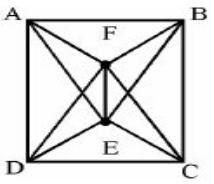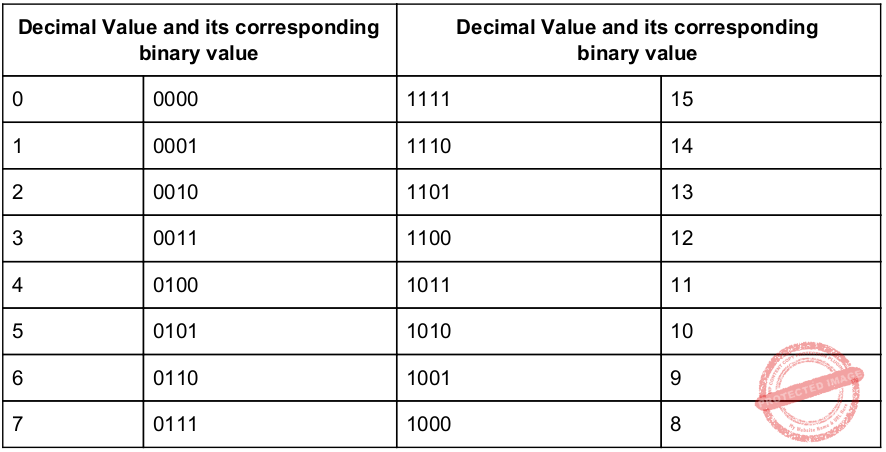## UGC NET CS 2014 Dec-Paper-2

 Question 1
Consider a set A = {1, 2, 3, ........, 1000}. How many members of A shall be divisible by 3 or by 5 or by both 3 and 5 ?
 A 533 B 599 C 467 D 66
Engineering-Mathematics       Probability
Question 1 Explanation:
Method-1:
Given data,
-- Set A={1, 2, 3, ........, 1000}
-- Set A shall be divisible by 3=?
-- Set A shall be divisible by 5=?
-- Set A shall be divisible by 3 and 5=?
Step-1: To find divisible by 3 numbers are
=⌊1000/3⌋
= 333
Step-2: To find divisible by 5 numbers are
=⌊1000/5⌋
= 200
Step-3: To find divisible by 3 and 5 numbers are
=⌊1000/(3*5)⌋
= 66
These 66 is already part of 333 and 200. So, we have to exclude it from the list.
Total= 333+200-66
= 467
Note: We are using floor because excluding fraction value.
Method-2:
The above problem is in the form of (AUB) = (A)+(B)-(A∩B)
A=1000/3
B=1000/5
(A∩B)=66
(AUB)=467
Note: Getting this idea in exam hall is very difficult. So, better follow method-1 ratherhan method-2
 Question 2
A certain tree has two vertices of degree 4, one vertex of degree 3 and one vertex of degree 2. If the other vertices have degree 1, how many vertices are there in the graph ?
 A 5 B n-3 C 20 D 11
Engineering-Mathematics       Graph-Theory
Question 2 Explanation:
Method-1:
Here, they are clearly mentioned that “certain tree”
The tree contain maximum n-1 edges. Here, ‘n’ is number of vertices.
→ According to the handshaking lemma “sum of degrees of all vertices=2|e|”.
→ Two vertices of degree 4, we can write into (2*4)
→ One vertex of degree 3, we can write into (1*3)
→ One vertex of degree 2, we can write into (1*2).
→ Other vertices(X) have degree 1, we can write into (X*1)
Step-1: (2*4)+(1*3)+(1*2)+(X*1)
=2(X+4-1) [Note: ‘X’ value is not given ]
X=7
Step-2: To find total number of vertices, we are adding X+4 because they already given 4 vertices.
=X+4
=7+4
=11
Method-2:
Draw according to given constraints but it not suitable for very big treesB, C, E, F, H, I, J degree = 1
D degree = 2
A degree = 4
K degree = 4
G degree = 3
 Question 3
Consider the Graph shown below :This graph is a __________.
 A Complete Graph B Bipartite Graph C Hamiltonian Graph D All of the above
Engineering-Mathematics       Graph-Theory
Question 3 Explanation:
Option-A: It is not complete graph because it won’t have n(n-1)/2 edges.
Option-B: It is not Bipartite Graph because it takes more than 2 colours. Bipartite Graph have exactly 2 colours.
Option-C: Hamiltonian path is a path in an undirected or directed graph that visits each vertex exactly once.Question 4
A computer program selects an integer in the set {k : 1 ≤ k ≤ 10,00,000} at random and prints out the result. This process is repeated 1 million times. What is the probability that the value k=1 appears in the printout at least once ?
 A 0.5 B 0.704 C 0.632121 D 0.68
Engineering-Mathematics       Probability
Question 4 Explanation:
Probability that the value k=1 appears in the printout at least once is
1-p(x=0)
We will apply the formula of binomial distribution
1-(​ 1000000​ C​ 0​ (1/1000000)​ 0​ * (999999/1000000)​ 10^6​ )
=1-(1*1*0.367879)
=0.632121
 Question 5
If we define the functions f, g and h that map R into R by : f(x) = x​ 4​ , g(x) = √ x 2 + 1 , h(x) = x​ 2​ + 72, then the value of the composite functions ho(gof) and (hog)of are given as
 A x​ 8​ – 71 and x​ 8​ – 71 B x​ 8​ – 73 and x​8​ – 73 C x​ 8​ + 71 and x​ 8​ + 71 D x​ 8​ + 73 and x​ 8​ + 73
Engineering-Mathematics       Functions
Question 5 Explanation:
Given f(x) = x​ 4​ , g(x) = √ x 2 + 1 , h(x) = x​ 2​ + 72
for, ho(gof)
gof=g(f(x))
=g(x​ 4​ )
=√(x​ 8​ +1)
ho(gof)=h(gof)
=h(√(x​ 8​ +1))
=(√(x​ 8​ +1)​ 2​ +72
=x​ 8​ +1+72
=x​ 8​ +73
√ x 2 + 1 , h(x) = x​ 2​ + 72
for, (hog)of,
hog=h(g(x))
=h(√(x​ 2​ +1)
=(√(x​ 2​ +1)​ 2​ +72
=x​ 2​ + 1+72
=x​ 2​ +73
(hog)of=(hog)(f(x))
=(hog)(x​ 4​ )
=(x​ 4​ )​ 2​ +73
=x​ 8​ +73
Hence, option-D is the correct answer
 Question 6
Digital-Logic-Design       Number-Systems
Question 6 Explanation:
→ Each digit is represented by a 4-bit BCD code.
→ To add two 4-bit number, we need 1 Half Adder(to add LSBs) and 3 Full Adders(remaining three bits of both number along with carry bits).
→ To make the resultant Sum as valid BCD sum, we need to add 0110 to the sum.
→ This can be done with 1 Half adder and 2 Full Adder
(Note: LSB bit of 0110 is always zero. So there is no need of ADDER to add LSBs.)
 Question 7
The Excess-3 decimal code is a self-complementing code because
 A The binary sum of a code and its 9’s complement is equal to 9. B It is a weighted code C Complement can be generated by inverting each bit pattern D The binary sum of a code and its 10’s complement is equal to 9
Digital-Logic-Design       Number-Systems
Question 7 Explanation:
The Excess-3 decimal code is a self-complementing code because complement can be generated by inverting each bit pattern.Question 8
How many PUSH and POP operations will be needed to evaluate the following expression by reverse polish notation in a stack machine (A * B) + (C * D/E) ?
 A 4 PUSH and 3 POP instructions B 5 PUSH and 4 POP instructions C 6 PUSH and 2 POP instructions D 5 PUSH and 3 POP instructions
Data-Structures       Queues-and-Stacks
Question 8 Explanation:
Given infix notation, we have to change infix notation into postfix notation.
after converting postfix notation the notations are ab* cde /* +Total 5 PUSH and 4 POP operations performed.
 Question 9
The range of representable normalized numbers in the floating point binary fractional representation in a 32-bit word with 1-bit sign, 8-bit excess 128 biased exponent and 23-bit mantissa is
 A 2​ -128​ to (1 – 2​ –23​ ) * 2​ 127 B (1 – 2​ –23​ ) * 2​ ​ -127 ​ to 2​ 128 C (1 – 2​ –23​ ) * 2​ –127​ to 2​ 23> D 2​ –129​ to (1 – 2​ –23​ ) * 2​ 127
Digital-Logic-Design       Number-Systems
Question 9 Explanation:
The range of representable normalized numbers in the floating point binary fractional representation in a 32-bit word with 1-bit sign, 8-bit excess 128 biased exponent and 23-bit mantissa is​ ​ 2 –129 to (1 – 2​ –23​ ) * 2​ 127
 Question 10
The size of the ROM required to build an 8-bit adder/subtractor with mode control, carry input, carry output and two’s complement overflow output is given as
 A 2​ 16​ * 8 B 2​ 18​ * 10 C 2​ 16​ * 10 D 2​ 18​ * 8
Computer-Organization       ROM
Question 10 Explanation:
The size of the ROM required to build an 8-bit adder/subtractor with mode control, carry input, carry output and two’s complement overflow output is given as 2​ 18​ * 10.
 Question 11
What will be the output of the following ‘C’ code ?
main( )
{
int x=128;
printf (“\n%d”, 1 + x++);
}
 A 128 B 129 C 130 D 131
Programming       Programming
Question 11 Explanation:
In this program we are using post increment. Post increment will send the value before updating the value.
printf(“\n%d”, 1 + x++); /* x=128 */
Here, 1+128=129
 Question 12
What does the following expression means ? char *(*(*a[N]) ( )) ( );
 A a pointer to a function returning array of n pointers to function returning character pointers. B a function return array of N pointers to functions returning pointers to characters C an array of n pointers to function returning pointers to characters D an array of n pointers to function returning pointers to functions returning pointers to characters.
Programming       Programming
Question 12 Explanation:
char *(*(*a[N]) ( )) ( ); /* an array of n pointers to function returning pointers to functions returning pointers to characters */
There are 12 questions to complete.

Register Now